## Convert a Referenced Model to Fixed Point

When a system under design contains a referenced model, the Fixed-Point Tool proposes data types for the objects in the referenced model based on ranges collected through simulation or derived range analysis. If the system under design contains several instances of the same referenced model, the Fixed-Point Tool uses a union of the collected ranges for data type proposals.

The Fixed-Point tool logs simulation minimum and maximum values only for instances of the referenced model that are in normal mode. It does not log simulation minimum and maximum values for instances of the referenced model that are in non-Normal modes. If your model contains multiple instances of a referenced model and some are instances are in normal mode and some are not, the tool logs and displays data for those that are in normal mode.

Open the `ex_mdlref_controller` model. At the MATLAB® command line, enter:

```addpath(fullfile(docroot,'toolbox','fixpoint','examples')); ex_mdlref_controller```

In the Apps gallery of the model, select Fixed-Point Tool.

When a model contains a referenced model, the Fixed-Point Tool Model Hierarchy pane displays a subnode for the instance of the referenced model and a node for the referenced model. For example, the `ex_mdlref_controller` model contains a Model block that references the `ex_controller` model. The Fixed-Point Tool shows both models in the model hierarchy.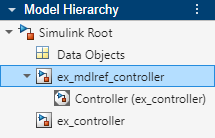If a model contains multiple instances of a referenced model, the tool displays each instance of the referenced model in this model and a node for the referenced model. For example, in the same model, if you duplicate the referenced model such that the `ex_mdlref_controller` model contains two instances of the referenced model `ex_controller`. The Fixed-Point Tool displays both models and both instances of the referenced model in the model hierarchy.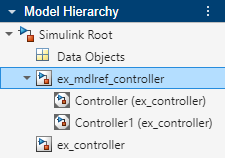### Viewing Simulation Ranges for Referenced Models

1. In the Fixed-Point Tool, under New, select the `Iterative Fixed-Point Conversion` workflow.

2. Under System Under Design (SUD), select the `ex_controller` model as the system you want to convert to fixed point.

3. Under Range Collection Mode, select as the range collection method.

4. In the toolstrip, click . The Fixed-Point Tool checks the system under design for compatibility with the conversion process and reports any issues found in the model. In this example, the tool reports that the model is ready for conversion.

5. Expand the button arrow and select `Double precision`. Click the button to start the simulation. The Fixed-Point Tool overrides the data types in the model with doubles and collects the minimum and maximum values for each object in your model that occur during the simulation. The Fixed-Point Tool stores this range information in a run titled `BaselineRun`.

The tool logs and displays the results for each instance of the referenced model. For example, here are the results for the first instance of the referenced model `ex_controller`.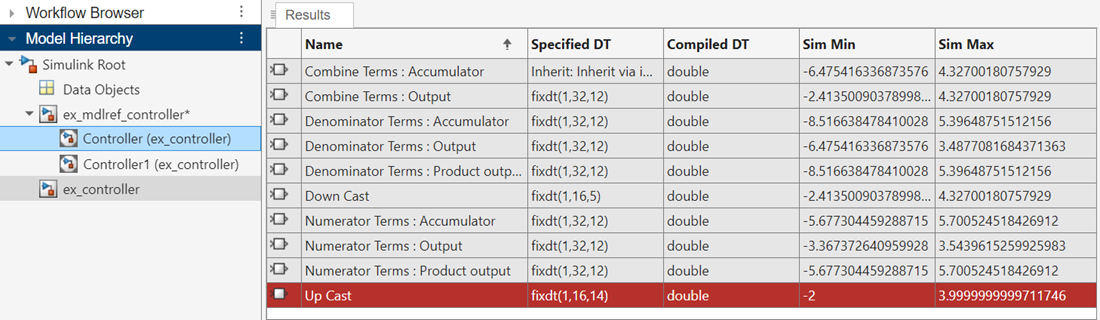Here are the results for the second instance of `ex_controller`.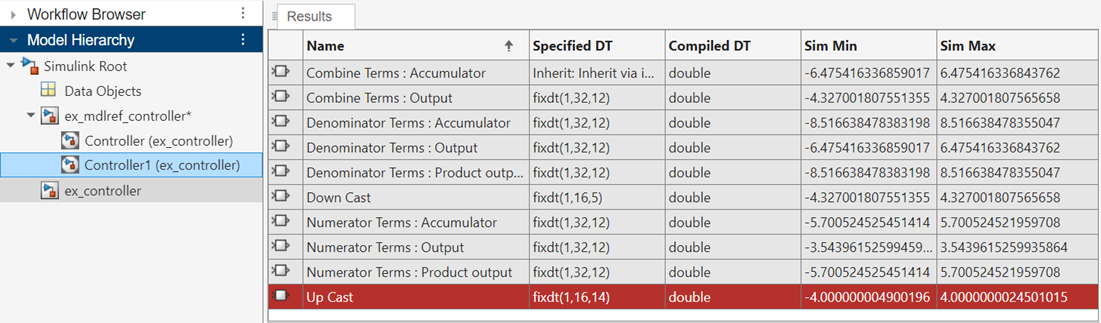In the referenced model node, the tool displays the union of the results for each instance of the referenced model.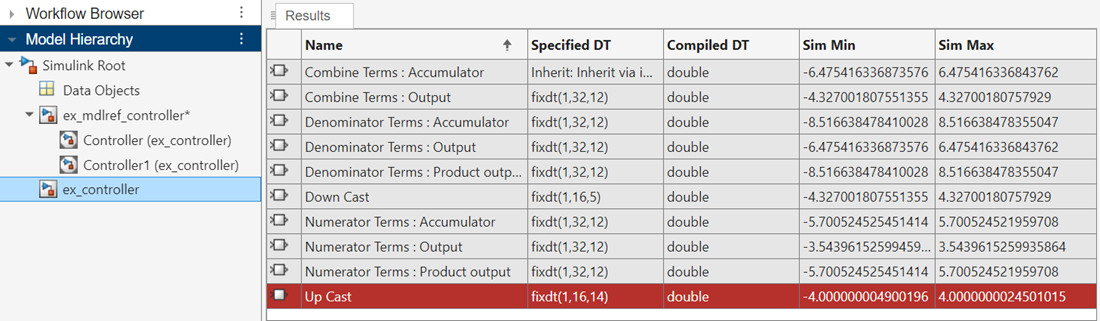#### Fixed-Point Instrumentation and Data Type Override Settings

When you simulate a model that contains referenced models, the data type override and fixed-point instrumentation settings for the top-level model do not control the settings for the referenced models. You must specify these settings separately for the referenced model. If the settings are inconsistent, for example, if you set the top-level model data type override setting to double and the referenced model to use local settings and the referenced model uses fixed-point data types, data type propagation issues might occur.

When you change the fixed-point instrumentation and data type override settings for any instance of a referenced model, the settings change on all instances of the model and on the referenced model itself.

### Propose Data Types for a Referenced Model

1. In the Convert section of the toolstrip, click Settings. Specify the Safety margin for simulation min/max (%) parameter as `20`.

2. Click .

Because no design minimum and maximum information is supplied, the simulation minimum and maximum data that was collected during the simulation run is used to propose data types. The Safety margin for simulation min/max (%) parameter value multiplies the “raw” simulation values by a factor of 1.2. Setting the Safety margin for simulation min/max (%) parameter to a value greater than 1 decreases the likelihood that an overflow will occur when fixed-point data types are being used.

Because of the nonlinear effects of quantization, a fixed-point simulation produces results that are different from an idealized, doubles-based simulation. Signals in a fixed-point simulation can cover a larger or smaller range than in a doubles-based simulation. If the range increases enough, overflows or saturations could occur. A safety margin decreases the likelihood of this happening, but it might also decrease the precision of the simulation.

The Fixed-Point Tool analyzes the scaling of all fixed-point blocks whose Lock output data type setting against changes by the fixed-point tools parameter is not selected.

The Fixed-Point Tool uses the minimum and maximum values collected during simulation to propose a scaling for each block such that the precision is maximized while the full range of simulation values is spanned. The tool displays the proposed scaling in the spreadsheet.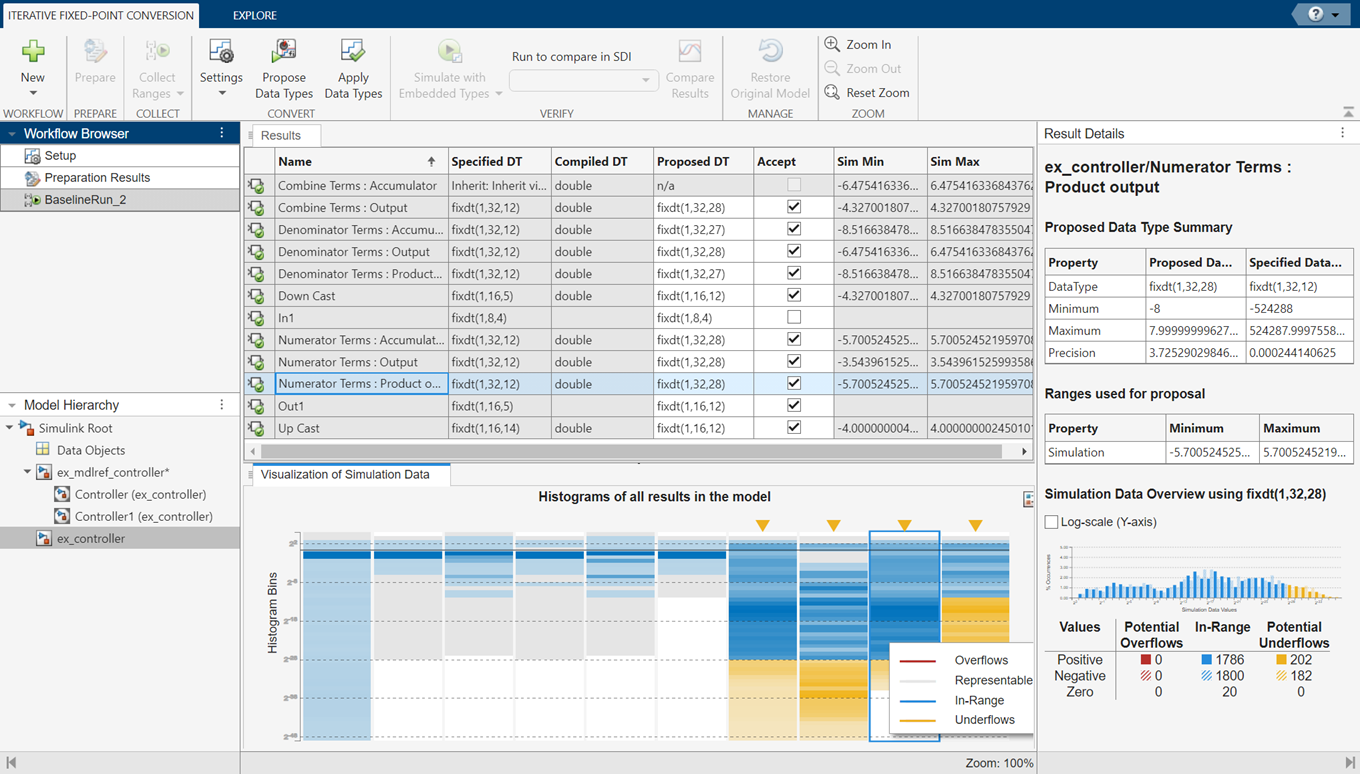3. Review the scaling that the Fixed-Point Tool proposes. You can choose to accept the scaling proposal for each block by selecting the corresponding Accept check box. By default, the Fixed-Point Tool accepts all scaling proposals that differ from the current scaling. For this example, verify that the Accept check box is selected for each of the Controller system’s blocks.

4. In the Fixed-Point Tool, click the button.

The Fixed-Point Tool applies the scaling proposals that you accepted in the previous step.

5. In the Verify section of the toolstrip, click the button.

Simulink® simulates the `ex_mdlref_controller` model using the new scaling that you applied. Afterward, the Fixed-Point Tool displays information about blocks that logged fixed-point data.

6. Click . The Simulation Data Inspector plots the Analog Plant output for the floating-point and fixed-point runs and the difference between them.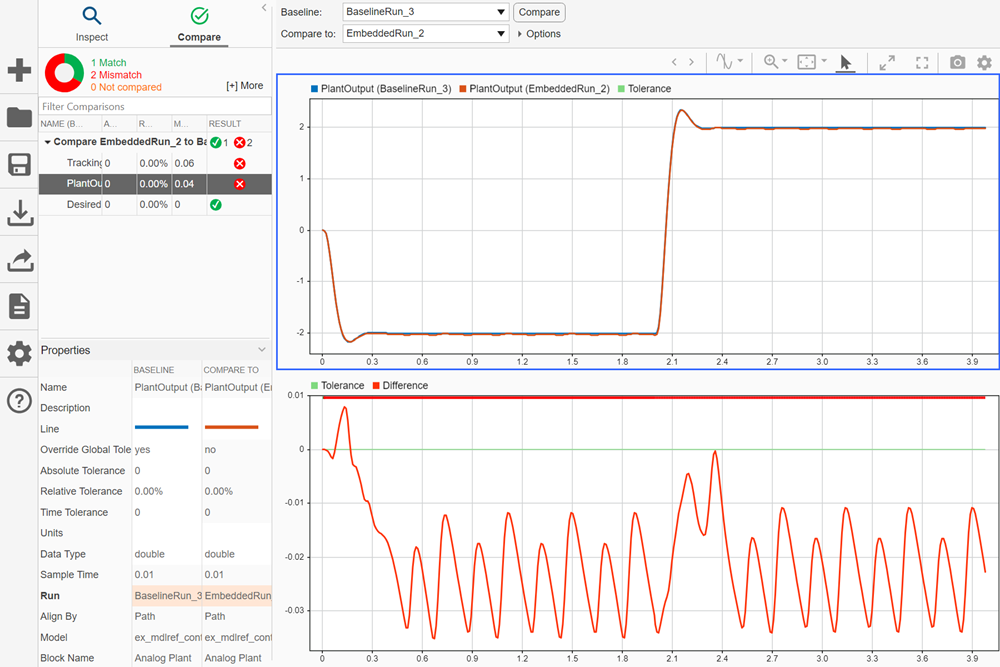## SupportGet trial now